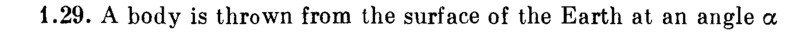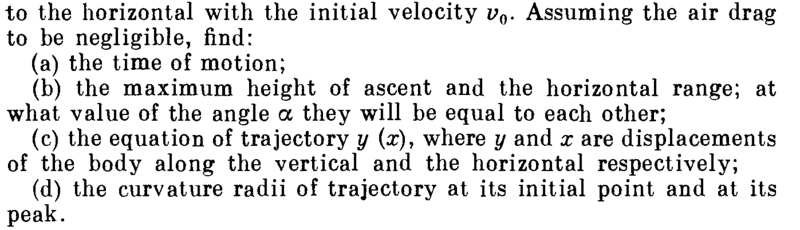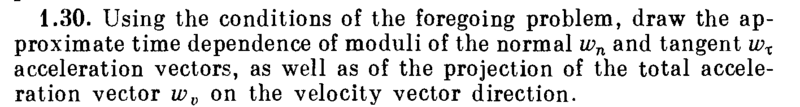# Projectile motion, radii of trajectory

## Homework Statement## The Attempt at a Solution

For part (d),
The curvature radius of trajectory at its
1) initial point = horizontal range/2 =( v02 sin (2α))/2g
2) peak = height of ascent/2 = ( v0 sinα)2 /2g

Is this correct?

In this problem, the time of ascent is equal to the time of descent.
Is there anyway to find it out without calculating the time of ascent and the time of descent ?vy (t) = v0 sinα - gt
vx (t) = v0 cosα

tan (θ) = vy (t) / vx (t) = tan α - gt/(v0 cosα)

a = g(- ## \hat y##)
wτ = g sinθ (- ## \hat θ##),
wn = g cosθ (- ## \hat r##)

Is this correct so far?

Last edited:
cnh1995
Homework Helper
Gold Member
In this problem, the time of ascent is equal to the time of descent.
Is there anyway to find it out without calculating the time of ascent and the time of descent ?
You can put (vertical) displacement=0 in the y-displacement equation. It's a quadratic equation.

You can put (vertical) displacement=0 in the y-displacement equation. It's a quadratic equation.
This will give me the total time of motion and this, too, I will have to calculate.
I want to show :
time of ascent = time of descent without doing calculation, just on the basis of physical interpretation of the problem
Is this possible?

cnh1995
Homework Helper
Gold Member
time of ascent = time of descent without doing calculation, just on the basis of physical interpretation of the problem
Is this possible?
Yes.
Your x component of velocity doesn't change. And during ascent, you start with non-zero velocity and end up with zero velocity while during descent, you start with zero velocity and end up with the initial velocity. Displacement is same in both the cases and the motion is under the influence of the same force, i.e. gravity.
The time a body takes to go up to a certain height is equal to the time it takes to free fall from the same height.

cnh1995
Homework Helper
Gold Member
) initial point = horizontal range/2 =( v02 sin (2α))/2g
2) peak = height of ascent/2 = ( v0 sinα)2 /2g
You need to consider the centripetal forces responsible for the curvature here.
Which force is responsible for the curvature when the body is at the peak? How much is that force?

You need to consider the centripetal forces responsible for the curvature here.
Which force is responsible for the curvature when the body is at the peak? How much is that force?

When the body is at the initial point,

mv02 /Ri = mg cosα
Ri = v02/g cosα

When the body is at the peak,
m(v0 cosα)2 /Rp = mg
Rp = (v0 cosα)2/g

Is this correct?

cnh1995
Homework Helper
Gold Member
When the body is at the initial point,

mv02 /Ri = mg cosα
Ri = v02/g cosα

When the body is at the peak,
m(v0 cosα)2 /Rp = mg
Rp = (v0 cosα)2/g

Is this correct?
Yes.

What about the next question given in post 2?

cnh1995
Homework Helper
Gold Member
a = g(- ^yy^ \hat y)
wτ = g sinθ (- ^θθ^ \hat θ),
wn = g cosθ (- ^rr^ \hat r)
Correct. So now you can draw the approximate plot of the tangential and normal acceleration.
You know their values at t=0, t=T/2 and t=T.